### Playing With Numbers Class 8th Mathematics AP Board Solution

##### Question 1.Find the sum of integers which are divisible by 5 from 1 to 100.Answer:Given, Sum of integers from 1 to 100Need to find out the numbers which are divisible by 5.The numbers which are divisible by 5 are 5,10,15…..100⇒ Now, Sum of integers form 1 to N =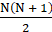Sum of integers from 1 to 100 divisible by 5= (5 + 10 + ….. + 100)= 5(1 + 2 + …. + 20)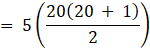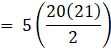= (5 × 10 × 21)= 1050Hence, the sum of integers which are divisible by 5 from 1 to 100 is 1050.Question 2.Find the sum of integers which are divisible by 2 from 11 to 50.Answer:Given, Sum of integers from 11 to 50we need to find out the numbers which are divisible by 2⇒ Now, Sum of integers form 1 to N =⇒ Sum of integers divisible by 2 from 11 to 50 = Sum of integers divisible by 2 from 1 to 50 – Sum of integers divisible by 2 from 1 to 49= (2 + 4 + 6………. + 50) – (2 + 4 + 6…. + 10)= 2(1 + 2 + 3…… + 25)-2(1 + 2 + .. + 5)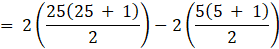= (25 × 26)-(5 × 6)= 650 – 30= 620Hence, the sum of integers which are divisible by 2 from 11 to 50 is 620Question 3.Find the sum of integers which are divisible by 2 and 3 from 1 to 50.Answer:Given, Sum of integers from 1 to 50Need to find out the numbers which are divisible by 2 and 3.Here, since the number should be divisible by 2 and also 3 it is enough to find the sum of integers which are divisible by 6.∴ the sum of numbers which are divisible by 6 from 1 to 50 = 6,12,18…48⇒ Now, Sum of integers form 1 to N == (6 + 12 + 18 + ….. + 48)= 6(1 + 2 + 3 + …. + 8)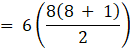= 3(8 × 9)= 216Hence, the sum of integers from 1 to 50 which are divisible by 2 and 3 is 216.Question 4.(n3 − n) is divisible by 3. Explain the reason.Answer:Given, (n3 – n) is divisible by 3Here, (n3 – n) can be written as n(n2 -1)⇒ n(n2 -12)= n(n-1)(n + 1)= (n-1)n(n + 1)It is the product of three consecutive numbersThe product of three consecutive numbers is divisible by 3Example:Let n = 2Substitute in the above equation we get⇒ (2-1)(2)(2 + 1)= 1 × 2 × 3= 6 which is divisible by 3Hence, (n3 – n) is divisible by 3Question 5.Sum of ‘n’ odd number of consecutive numbers is divisible by ‘n’. Explain the reason.Answer:Given, sum of ‘n’ odd number of consecutive numbers is divisible by ‘n’.Sum of n consecutive odd numbers =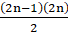= n(2n-1)∴ it is multiple of nHence, sum of n consecutive odd numbers is divisible by nQuestion 6.Is 111 + 211 + 311 + 411 divisible by 5? Explain.Answer:Given, 111 + 211 + 311 + 411We need to find out the given number is divisible by 5No, the given number cannot be divisible by 5Explanation:For a number to be divisible by 5, it should end with 0 or 5.Hence, the given number cannot be divisible by 5Question 7.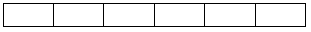Find the number of rectangles of the given figure?Answer:Given, a rectangle gridWe need to find out the number of rectangles in the figure⇒ 1 + 2 + 3 + 4 + 5 + 6 = 21Hence, 21 rectangles are there in the given figure.Question 8.Rahul’s father wants to deposit some amount of money every year on the day of Rahul’s birthday. On his 1st birth day Rs.100, on his 2nd birth day Rs.300, on his 3rd birth day Rs.600, on his 4th birthday Rs.1000 and so on. What is the amount deposited by his father on Rahul’s 15th birthday.Answer: Money deposited by Rahul's father is as follows:100, 300, 600, 1000They are in following series100 + 1 × 0, 100 + 2 × 100, 300 + 3 × 100, 600 + 4 × 100Multiplication numbers 1, 2, 3 & 4 represent the age Rahul is turning. The multiplication factor is previous year deposit.If we say, n is number of years, Rahul is turning and T1 = 100. Then the series can be re written as (T0 = 0)T1 + n × T0 , T2 = T1 + n × 100, T3 = T2 + n × 100 = T1 + (n-1) × 100 + n × 100T4 = T3 + n × 100 = T1 + (n - 2) × 100 + (n - 1) × 100 + n × 100....T15 = T14 + 15 × 100= 1 × 100 + 2 × 100 + 3 × 100 + ........+ 15 × 100All the terms after T1 are in AP where, nth term is sum of n terms of the AP wherea = 100 and d = 100 n = 15Hence T15 = = n(2a + (n-1)d)/2= 15(200 + 14 × 100)/2= 15 × 800= 12000Question 9.Find the sum of integers from 1 to 100 which are divisible by 2 or 5.Answer:Given, Sum of integers from 1 to 100Need to find out the numbers which are divisible by 2 or 5.⇒The numbers which are divisible by 2 are 2,4,6….100And the numbers which are divisible by 5 are 5,10,15…..100⇒ Sum numbers are repeated twice hence, we need to take the LCM of 2 and 5 which is 10∴ sum of numbers which are divisible by 10 are 10,20……100⇒ Now, Sum of integers form 1 to N =∴ Sum of integers divisible by 2 or 5 from 1 to 100 = Sum of integers from 1 to 100 divisible by 2 + Sum of integers from 1 to 100 divisible by 5 – Sum of integers from 1 to 100 divisible by 10= (2 + 4….. + 100) + (5 + 10 + ….. + 100)-(10 + 20 + …… + 100)= 2(1 + 2 + … + 50) + 5(1 + 2 + …. + 20)-10(1 + 2 + ….. + 10)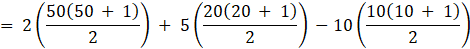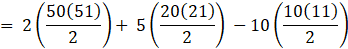= (50 × 51) + (5 × 10 × 21)-(5 × 10 × 11)= 2550 + 1050-550= 3050Hence, the sum of integers from 1 to 100 which are divisible by 2 or 5 is 3050Question 10.Find the sum of integers from 11 to 1000 which are divisible by 3.Answer:Given, Sum of integers from 11 to 1000we need to find out the numbers which are divisible by 3⇒ Now, Sum of integers form 1 to N =⇒ Sum of integers divisible by 3 from 11 to 1000 = Sum of integers divisible by 3 from 1 to 1000 – Sum of integers divisible by 3 from 1 to 10= (3 + 6 + 9………. + 999) – (3 + 6 + …. + 9)= 3(1 + 2 + 3…… + 333)-3(1 + 2 + .. + 3)=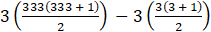=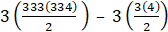= 3(333(167)) – 3(6)= 3(55611)-18= 166833-18= 166815Hence, the sum of integers from 11 to 1000 which are divisible by 3 is 166815.

PDF FILE TO YOUR EMAIL IMMEDIATELY PURCHASE NOTES & PAPER SOLUTION. @ Rs. 50/- each (GST extra)

HINDI ENTIRE PAPER SOLUTION

MARATHI PAPER SOLUTION

SSC MATHS I PAPER SOLUTION

SSC MATHS II PAPER SOLUTION

SSC SCIENCE I PAPER SOLUTION

SSC SCIENCE II PAPER SOLUTION

SSC ENGLISH PAPER SOLUTION

SSC & HSC ENGLISH WRITING SKILL

HSC ACCOUNTS NOTES

HSC OCM NOTES

HSC ECONOMICS NOTES

HSC SECRETARIAL PRACTICE NOTES

# 2019 Board Paper Solution

HSC ENGLISH SET A 2019 21st February, 2019

HSC ENGLISH SET B 2019 21st February, 2019

HSC ENGLISH SET C 2019 21st February, 2019

HSC ENGLISH SET D 2019 21st February, 2019

SECRETARIAL PRACTICE (S.P) 2019 25th February, 2019

HSC XII PHYSICS 2019 25th February, 2019

CHEMISTRY XII HSC SOLUTION 27th, February, 2019

OCM PAPER SOLUTION 2019 27th, February, 2019

HSC MATHS PAPER SOLUTION COMMERCE, 2nd March, 2019

HSC MATHS PAPER SOLUTION SCIENCE 2nd, March, 2019

SSC ENGLISH STD 10 5TH MARCH, 2019.

HSC XII ACCOUNTS 2019 6th March, 2019

HSC XII BIOLOGY 2019 6TH March, 2019

HSC XII ECONOMICS 9Th March 2019

SSC Maths I March 2019 Solution 10th Standard11th, March, 2019

SSC MATHS II MARCH 2019 SOLUTION 10TH STD.13th March, 2019

SSC SCIENCE I MARCH 2019 SOLUTION 10TH STD. 15th March, 2019.

SSC SCIENCE II MARCH 2019 SOLUTION 10TH STD. 18th March, 2019.

SSC SOCIAL SCIENCE I MARCH 2019 SOLUTION20th March, 2019

SSC SOCIAL SCIENCE II MARCH 2019 SOLUTION, 22nd March, 2019

XII CBSE - BOARD - MARCH - 2019 ENGLISH - QP + SOLUTIONS, 2nd March, 2019

# HSCMaharashtraBoardPapers2020

(Std 12th English Medium)

HSC ECONOMICS MARCH 2020

HSC OCM MARCH 2020

HSC ACCOUNTS MARCH 2020

HSC S.P. MARCH 2020

HSC ENGLISH MARCH 2020

HSC HINDI MARCH 2020

HSC MARATHI MARCH 2020

HSC MATHS MARCH 2020

# SSCMaharashtraBoardPapers2020

(Std 10th English Medium)

English MARCH 2020

HindI MARCH 2020

Hindi (Composite) MARCH 2020

Marathi MARCH 2020

Mathematics (Paper 1) MARCH 2020

Mathematics (Paper 2) MARCH 2020

Sanskrit MARCH 2020

Sanskrit (Composite) MARCH 2020

Science (Paper 1) MARCH 2020

Science (Paper 2)

Geography Model Set 1 2020-2021

MUST REMEMBER THINGS on the day of Exam

Are you prepared? for English Grammar in Board Exam.

Paper Presentation In Board Exam

How to Score Good Marks in SSC Board Exams

Tips To Score More Than 90% Marks In 12th Board Exam

How to write English exams?

How to prepare for board exam when less time is left

How to memorise what you learn for board exam

No. 1 Simple Hack, you can try out, in preparing for Board Exam

How to Study for CBSE Class 10 Board Exams Subject Wise Tips?

JEE Main 2020 Registration Process – Exam Pattern & Important Dates

NEET UG 2020 Registration Process Exam Pattern & Important Dates

How can One Prepare for two Competitive Exams at the same time?

8 Proven Tips to Handle Anxiety before Exams!